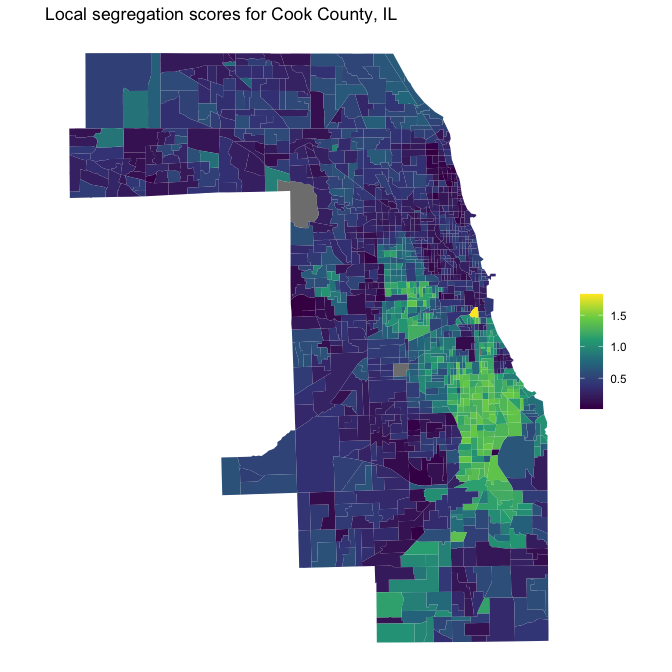# FAQ

## Can index X be added to the package?

Adding new segregation indices is not a big trouble. Please open an issue on GitHub to request an index to be added.

## How can I compute indices for different areas at once?

If you use the dplyr package, one pattern that works well is to use group_modify. Here, we compute the pairwise Black-White dissimilarity index for each state separately:

library("segregation")
library("dplyr")

schools00 %>%
filter(race %in% c("black", "white")) %>%
group_by(state) %>%
group_modify(~ dissimilarity(
data = .x,
group = "race",
unit = "school",
weight = "n"
))
#> # A tibble: 3 × 3
#> # Groups:   state 
#>   state stat    est
#>   <fct> <chr> <dbl>
#> 1 A     D     0.706
#> 2 B     D     0.655
#> 3 C     D     0.704

A similar pattern works also well with data.table:

library("data.table")

schools00 <- as.data.table(schools00)
schools00[
race %in% c("black", "white"),
dissimilarity(data = .SD, group = "race", unit = "school", weight = "n"),
by = .(state)
]
#>     state   stat       est
#>    <fctr> <char>     <num>
#> 1:      A      D 0.7063595
#> 2:      B      D 0.6548485
#> 3:      C      D 0.7042057

To compute many decompositions at once, it’s easiest to combine the data for the two time points. For instance, here’s a dplyr solution to decompose the state-specific M indices between 2000 and 2005:

# helper function for decomposition
diff <- function(df, group) {
data1 <- filter(df, year == 2000)
data2 <- filter(df, year == 2005)
mutual_difference(data1, data2, group = "race", unit = "school", weight = "n")
}

schools00$year <- 2000 schools05$year <- 2005
combine <- bind_rows(schools00, schools05)

combine %>%
group_by(state) %>%
group_modify(diff) %>%
#> # A tibble: 5 × 3
#> # Groups:   state 
#>   state stat          est
#>   <fct> <chr>       <dbl>
#> 1 A     M1         0.409
#> 2 A     M2         0.445
#> 3 A     diff       0.0359
#> 5 A     removals   0.0390

Again, here’s also a data.table solution:

setDT(combine)
combine[, diff(.SD), by = .(state)] %>% head(5)
#>     state      stat         est
#>    <fctr>    <char>       <num>
#> 1:      A        M1  0.40859652
#> 2:      A        M2  0.44454379
#> 3:      A      diff  0.03594727
#> 5:      A  removals  0.03903106

## How can I use Census data from tidycensus to compute segregation indices?

Here are a few examples thanks to Kyle Walker, the author of the tidycensus package.

library("tidycensus")

cook_data <- get_acs(
geography = "tract",
variables = c(
white = "B03002_003",
black = "B03002_004",
asian = "B03002_006",
hispanic = "B03002_012"
),
state = "IL",
county = "Cook"
)
#> Getting data from the 2017-2021 5-year ACS

Because this data is in “long” format, it’s easy to compute segregation indices:

# compute index of dissimilarity
cook_data %>%
filter(variable %in% c("black", "white")) %>%
dissimilarity(
group = "variable",
unit = "GEOID",
weight = "estimate"
)
#>      stat       est
#>    <char>     <num>
#> 1:      D 0.7855711

# compute multigroup M/H indices
cook_data %>%
mutual_total(
group = "variable",
unit = "GEOID",
weight = "estimate"
)
#>      stat       est
#>    <char>     <num>
#> 1:      M 0.5114435
#> 2:      H 0.4089561

Producing a map of local segregation scores is also not hard:

library("tigris")
library("ggplot2")

local_seg <- mutual_local(cook_data,
group = "variable",
unit = "GEOID",
weight = "estimate",
wide = TRUE
)

seg_geom <- tracts("IL", "Cook", cb = TRUE, progress_bar = FALSE) %>%
left_join(local_seg, by = "GEOID")
#> Retrieving data for the year 2021

ggplot(seg_geom, aes(fill = ls)) +
geom_sf(color = NA) +
coord_sf(crs = 3435) +
scale_fill_viridis_c() +
theme_void() +
labs(
title = "Local segregation scores for Cook County, IL",
fill = NULL
)## How can I compute margins-adjusted local segregation scores?

When using mutual_difference, supply method = "shapley_detailed" to get two different local segregation scores that are margins-adjusted (one is coming from adjusting forward, the other from adjusting backwards). By averaging them we can create a single margins-adjusted local segregation score:

diff <- mutual_difference(schools00, schools05, "race", "school",
weight = "n", method = "shapley_detailed"
)

diff[stat %in% c("ls_diff1", "ls_diff2"),
by = .(school)
]
#> 1710: C167_1     -0.002663687